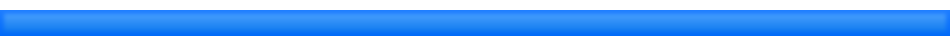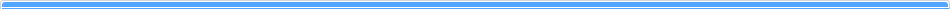﻿ Department of Mathematical Sciences, Shimane UniversityJapanese > TOP (English)

# Faculty members of Pure Mathematics

 Name Research field SUGIE Jitsuro Ordinary differential equations, function differential equations, difference equations and their applications to science NAKANISHI Tosihiro Complex analysis, in particular, the theory of Teichmuller spaces, hyperbolic geometry and discrete groups UEDA Akira Orders in Artinian rings, especially research on non-commutative valuation rings AOKI Miho Number theory. In particular, algebraic number theory and Iwasawa theory YAMADA Takumi Differential geometry MATSUHASHI Eiichi On approximation theorems of continuous maps and their applications WATANABE Tadayuki Differential topology, especially, invariants of manifolds and fiber bundles, and topological field theory MAETA Shun Differential Geometry, especially polyharmonic maps of order k and submanifolds# Faculty members of Applied Mathematics

 Name Research field KUROIWA Daishi Convex Analysis, Nonlinear Analysis, and Applications to Optimization Theory WADA Takeshi Partial differential equations, especially theory of well-posedness and asymptotic behavior of solutions to nonlinear dispersive equations SAITO Yasuhisa Ordinary differential/difference equations, delay differential equations, and mathematical biology, especially mathematics for population dynamics YAMADA Takayuki Mathematical Statistics SUZUKI Satoshi Nonlinear analysis and mathematical programming, especially research on quasiconvex programmingDepartment of Mathematical Sciences, Shimane University
■Tel:(0852) 32-6100　■ Fax:(0852) 32-6114